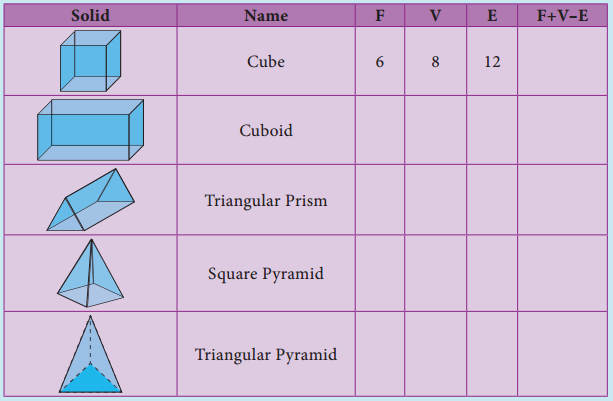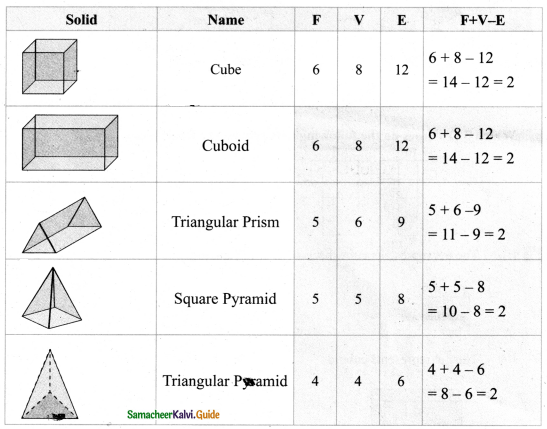# Samacheer Kalvi Books: Tamilnadu State Board Text Books Solutions

## Samacheer Kalvi 8th Maths Chapter 2 Measurements Notes PDF Download: Tamil Nadu STD 8th Maths Chapter 2 Measurements NotesSamacheer Kalvi 8th Maths Chapter 2 Measurements Notes PDF Download: Tamil Nadu STD 8th Maths Chapter 2 Measurements Notes

We bring to you specially curated Samacheer Kalvi 8th Maths Chapter 2 Measurements Notes PDF which have been prepared by our subject experts after carefully following the trend of the exam in the last few years. The notes will not only serve for revision purposes, but also will have several cuts and easy methods to go about a difficult problem.

 Board Tamilnadu Board Study Material Notes Class Samacheer Kalvi 8th Maths Subject 8th Maths Chapter Chapter 2 Measurements Format PDF Provider Samacheer Kalvi Books

## How to Download Samacheer Kalvi 8th Maths Chapter 2 Measurements Notes PDFs?

2. Click on the Samacheer Kalvi 8th Maths Notes PDF.
3. Look for your preferred subject.
4. Now download the Samacheer Kalvi 8th Maths Chapter 2 Measurements notes PDF.

## Tamilnadu Samacheer Kalvi 8th Maths Solutions Chapter 2 Measurements InText Questions

Think (Text Book Page No. 51)

Question 1.
227 and 3.14 are rational numbers. Is ‘π’ a rational number? Why?
227 and 3.14 are rational numbers π has non-terminating and non-repoating decimal expansion. So it is not a rational number. it is an irrational number.

Question 2.
When is the’π’day celebrated? Why?
March 14th i.e. 3/14 every year. Approximately value of’ ‘π’ is 3.14.

Think (Text Book Page No. 53)

The given circular figure is di4dd into six equal parts. Can we call the parts as sectors? Why?No, the equal parts are not sectors. Because a sector is a plane surface that is enclosed between two radii and the circular arc of the circle. Here the boundaries are not radii.

Try These (Text Book Page No. 53)

Fill the central angle of the shaded sector (each circle is divided into equal sectors)Think (Text Book Page No. 54)

Question 1.
Instead of multiplying by 1213 and 14, we shall multiply by 180360120360 and 90360 respectively. why?
So, 180360 = 12
120360 = 13
90360 = 14

Think (Text Book Page No. 57)

If the radius of a circle is doubled, what will happen to the area of the new circle so formed?
If r = 2r1 ⇒ Area of the circle = πr2 = π(2r1)2 = π4r12
Area = 4 × old area

Think (Text Book Page No. 61)

All the sides of a rhombus are equal. It is a regular polygon?
For a regular polygon all sides and all the angles must be equal. But in a rhombus all the sides are equal. But all the angles are not equal
∴ It is not a regular polygon.

Try This (Text Book Page No. 64)

In the above example split the given mat into two trapeziums and verify your answer.
Area of the mat = Area of I trapezium + Area of II trapezium
= [12 × h1 × (a1 + b1)] + [12 × h2 × (a2 + b2)] sq. units
= [12 × 2 × (7 + 5)] + 12 × 2 × (9 + 7) sq.feet
= 12 + 16 = 28 sq.feet
∴ Cost per sq.feet = ₹ 20
Cost for 28sq. feet = ₹ 20 × 28 = ₹ 560
∴ Total cost for the entire mat = ₹ 560
Both the answers are the same.

Try This (Text Book Page No. 68)

Tabulate the number of faces (F), vertices (V) and edges (E) for the following polyhedrons. Also find F + V – EWhat do you observe from the above table? We observe that, F + V – E = 2 in all the cases. This is true for any polyhedron and this relation F + V – E = 2 is known as Euler’s formula.From the table F + V – E = 2 for all the solid shapes.

## How to Prepare using Samacheer Kalvi 8th Maths Chapter 2 Measurements Notes PDF?

Students must prepare for the upcoming exams from Samacheer Kalvi 8th Maths Chapter 2 Measurements Notes PDF by following certain essential steps which are provided below.

• Use Samacheer Kalvi 8th Maths Chapter 2 Measurements notes by paying attention to facts and ideas.
• Pay attention to the important topics
• Refer TN Board books as well as the books recommended.
• Correctly follow the notes to reduce the number of questions being answered in the exam incorrectly
• Highlight and explain the concepts in details.

## Frequently Asked Questions on Samacheer Kalvi 8th Maths Chapter 2 Measurements Notes

#### How to use Samacheer Kalvi 8th Maths Chapter 2 Measurements Notes for preparation??

Read TN Board thoroughly, make separate notes for points you forget, formulae, reactions, diagrams. Highlight important points in the book itself and make use of the space provided in the margin to jot down other important points on the same topic from different sources.

#### How to make notes for Samacheer Kalvi 8th Maths Chapter 2 Measurements exam?

Read from hand-made notes prepared after understanding concepts, refrain from replicating from the textbook. Use highlighters for important points. Revise from these notes regularly and formulate your own tricks, shortcuts and mnemonics, mappings etc.
Share: